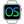• Overview
• Subtraction
• Comparison
WINDEVWEBDEVWINDEV MobileOthersOperations available for the dates, times and durations, ...
Overview
The following operations are available for the following types of variables: Date, Time, DateTime and Duration:
• subtraction
• comparison
Remark: Several WLanguage functions can be used to handle the dates and times (see the functions for managing the dates and times).
The addition operations performed between the Date, Time, DateTime and Duration variables are presented in the table below. This table presents the type of result for each combination:
Added typeDate Time DateTime Duration Date Concatenation of the elements into a string Concatenation of the elements into a string. This string can be used by DateTimeDifference Concatenation of the elements into a string Date variable (Time part ignored) Time Concatenation of the elements into a string Concatenation of the elements into a string Concatenation of the elements into a string Time variable (Date part ignored) DateTime Concatenation of the elements into a string Concatenation of the elements into a string Concatenation of the elements into a string DateTime variable Duration Date variable Time variable DateTime variable Duration variable
// Add a DateTime variable and a Time variable
StartDateTime is DateTime = "200112051530"
EndDateTime is DateTime = "200112051730"
ConferenceDuration is Duration = EndDateTime - StartDateTime

FinalDateTime is DateTime
// Add a DateTime variable and a Duration variable
dtMyDate is DateTime = DateSys() + TimeSys()
dtMyResultDate is DateTime

Trace("Start DateTime: " + dtMyDate)

StartTime is Time = "0117"
EndTime is Time = "2353"
duMyDuration is Duration = EndTime - StartTime
Trace("Duration in hours: " + NumToString(duMyDuration.InHours) + "h")

Trace("DateTime added to a duration: " + dtMyResultDate)
Subtraction
The subtraction operations performed between the Date, Time, DateTime and Duration variables are presented in the table below. This table presents the type of result for each combination:
Subtracted typeDate Time DateTime Duration Date Duration variable Error Error Date variable Time Error Duration variable Error Time variable DateTime Error Error Duration variable DateTime variable Duration Error Error Error Duration variable
StartDateTime is DateTime = "200112051530"
EndDateTime is DateTime = "200112071730"
ConferenceDuration is Duration = EndDateTime - StartDateTime
Comparison
The comparison operators (=, <>, <, >, <=, >=) used between the Date, Time, DateTime and Duration variables are presented in the table below. For each combination this table shows if the comparison is possible.
Compared typeDate Time DateTime Duration Date Possible Error Possible (Time part ignored) Error Time Error Possible Possible (Date part ignored) Error DateTime Possible (Time part ignored) Possible (Date part ignored) Possible Error Duration Error Error Error Possible
StartDateTime is DateTime = "200112051530"
EndDateTime is DateTime = "200115051730"
IF EndDateTime > StartDateTime THEN
Info("The dates are valid")
END
Minimum version required
• Version 9